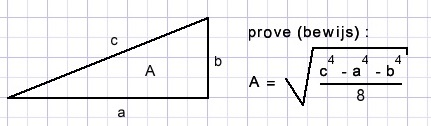Algebra problem collection (1..10)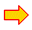problems 11..20

1.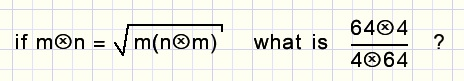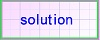2.
Simplify: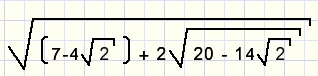3.
Calculate the radius of this circle: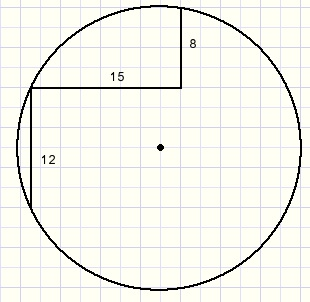4.Calculate angle x if c2 = a2 + 2 b25.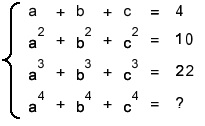6.
Prove:

 prove that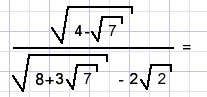17.

 givencalculate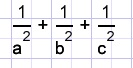8.
If x3 + 4x - 8 = 0 then calculate x7 + 64x29.

 ifthen calculate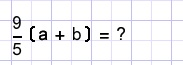10.
Prove the next formula for area A: